# Thread: Integration by Substitution

1. ## Integration by Substitution

Hello, I am having problem with this topic itself. I do not seem to understand the concept so here is a problem.

1.) p(p+1)^5 dp

2.) t/(t^2+2) dt

how do I go to solving these? I have tried many times and it just does not end up at the right spot, the definition in the book does not seem to explain well.
I make the brackets() = u and then find the derivitive of that and make it DU? but what do i do with the left over p and t? does that make sense?

Thanks

2. These are both nice examples of finding an anti-derivative by working backwards through the chain rule. So you see them both as the results of differentiation via the chain rule, and then try to get back to the starting points.

Remember that the chain rule produces a by-product, as it were... the derivative of the inner function. You differentiate with respect to the inner function, as though it were a variable, and then you multiply the result by the by-product - the derivative of the inner function.

So, for question 2, the whole of t^2 + 2 could well have been the inner function, so that the chain rule differentiation produced a by-product of 2t. So that would explain the t in the numerator. So the integral would seem to be... half of the ln of the inner function we just identified. Differentiate that, according to the chain rule, and you should get the integrand - try it.

u and du are a notation for tracing this process. You may see the point of them more easily for the first question. Anyway, I shall post a picture instead.

Edit:

Just in case a picture helps...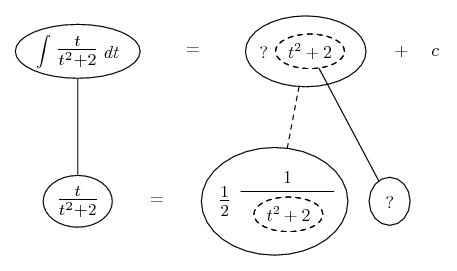... where... is the chain rule. Straight continuous lines differentiate downwards (integrate up) with respect to t, and the straight dashed line similarly but with respect to the dashed balloon expression (the inner function of the composite which is subject to the chain rule).

Spoiler: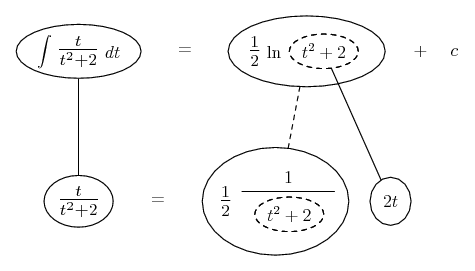Hope that helps. Will add similar for the other, anyway.

__________________________________________

Don't integrate - balloontegrate!

http://www.ballooncalculus.org/examples/gallery.html

http://www.ballooncalculus.org/asy/doc.html

3. hi and thank you,
but I still do not quite understand it....
so
u = t2 + 2
and du = 2t

how exactly do you end up with ln(t^2+2)/2?
or actually, how do you actually set the equation up in a u-du format?

du/u?

4.Originally Posted by cokeclassichi and thank you,
but I still do not quite understand it....
so
u = t2 + 2
and du = 2t

how exactly do you end up with ln(t^2+2)/2?
or actually, how do you actually set the equation up in a u-du format?

du/u?
You don't need to apply the substitution rule to evaluate $\displaystyle \int\frac{tdt}{t^2+2}$. You can just use the formula:

$\displaystyle \int\frac{f'(x)dx}{f(x)}=ln(f(x))+C$

Therefore:

$\displaystyle \frac{1}{2}\int\frac{2tdt}{t^2+2}=ln(t^2+2)+C$

The reason the formula works is because the derivative of $\displaystyle ln(f(x))+C$ is $\displaystyle \frac{f'(x)}{f(x)}$ by the chain rule.

5. I can't figure out how to apply the substitution rule to the integral $\displaystyle \int p(p+1)^5dp$. Maybe someone will come along with a clever substitution for that one, but are you sure you're suppose to apply the substitution rule? I haven't evaluated it yet, but I think integration by parts might be a more practical approach (that's just a hunch though). Are you familiar with that method?

6.Originally Posted by cokeclassichi and thank you,
but I still do not quite understand it....
so
u = t2 + 2
and du = 2t

how exactly do you end up with ln(t^2+2)/2?
or actually, how do you actually set the equation up in a u-du format?

du/u?

Well, I think the most fervant devotee of the u/du notation would prefer you at least to try doing without it at this stage, so you can see that the purpose of it is just (mainly) to work backwards through the chain rule. Have you tried applying the chain rule to differentiate the answer I've given you. You really should!

Edit: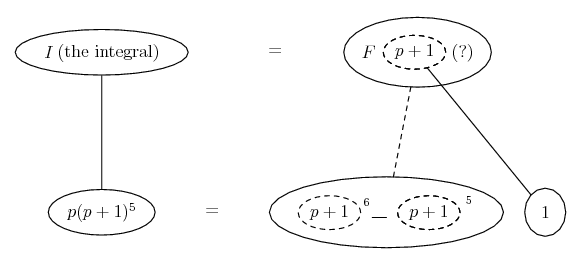For this one, we can treat p + 1 as the inner function. Notice the inner derivative is 1, so we can't, and don't need to, find some part of it in the integrand. Now, if we label the inner function u, as is the custom...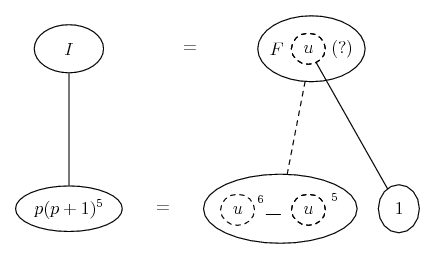... you might be happier about the lower equals sign...

$\displaystyle p + 1 = u\ \Rightarrow\ p(p + 1)^5 = (u - 1)u^5 = u^6 - u^5$

Spoiler: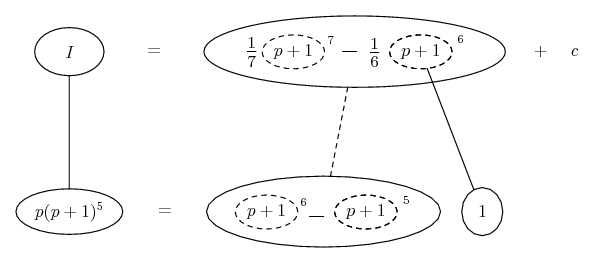__________________________________________

Don't integrate - balloontegrate!

http://www.ballooncalculus.org/examples/gallery.html

http://www.ballooncalculus.org/asy/doc.html

#### Search Tags

integration, substitution